Anderson mixing (AM) (Anderson, 1965) is a powerful acceleration method for fixed-point iterations. It extrapolates each new iterate using historical iterations. It is known that Anderson mixing is essentially equivalent to GMRES for solving linear systems (Walker & Ni, 2011). In the nonlinear case, it is recognized as a multi-secant quasi-Newton method (Fang & Saad, 2009) and has local linear convergence (Toth & Kelley, 2015).

Anderson mixing has several advantages, such as no additional functional evaluations and fast convergence in practice. However, one of the major concerns is the memory issue. Since the limited-memory method AM(m) uses $$m$$ historical iterations for extrapolation, the additional memory cost is $$2m$$ vector pairs of dimension $d$. Such memory cost can be prohibitive for solving high-dimensional problems in a resource-limited machine.

In our recent ICLR 2022 paper, we address the memory issue by developing the short-term recurrence Anderson mixing (ST-AM) methods, where only two additional vector pairs need to be stored, namely $$m=2$$. Our methods have the nature of quasi-Newton methods while the memory cost is largely reduced to be close to first-order methods. We validate the effectiveness of ST-AM in several applications including solving fixed-point problems and training neural networks.

## Anderson mixing

We first interpret Anderson mixing as a two-step procedure: (i) Projection step. The historical iterations are used to interpolate an intermediate iterate by solving a least squares problem. (ii) Mixing step. The current gradient is incorporated into the intermediate iterate.

AM is widely used to accelerate the slow convergence of fixed-point iterations in scientific computing, e.g., the self-consistent field iterations in electronic structure computations, full-waveform inversion. It is often found that AM can largely reduce the number of iterations to convergence. However, the memory overhead is a critical issue that prohibits the application of AM to large-scale and high-dimensional problems.

## Short-term recurrence Anderson mixing

We address the memory issue of AM by developing a class of short-term reccurence AM (ST-AM) methods. In the ST-AM methods, only two additional vector pairs need to be stored.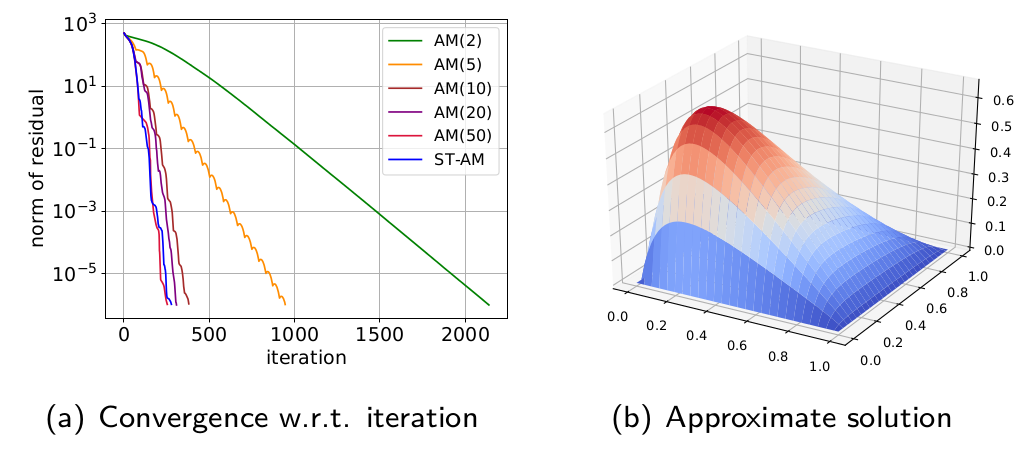The figures show applying AM, LBFGS, and ST-AM to solve partial differential equations (PDE). The first one is the convection-diffusion problem and the second one is the Bratu problem. The convergence behaviours suggest that ST-AM is very efficient for solving the traditional PDE problems in scientific computing.

The ST-AM methods consist of the basic ST-AM, the modified ST-AM and the regularized ST-AM.

### The basic ST-AM

By exploiting the symmetry of the Hessian in optimization, the basic ST-AM is equivalent to GMRES and the full-memory AM in strongly convex quadratic optimization. It also has linear convergence rate for solving nonsymmetric linear systems.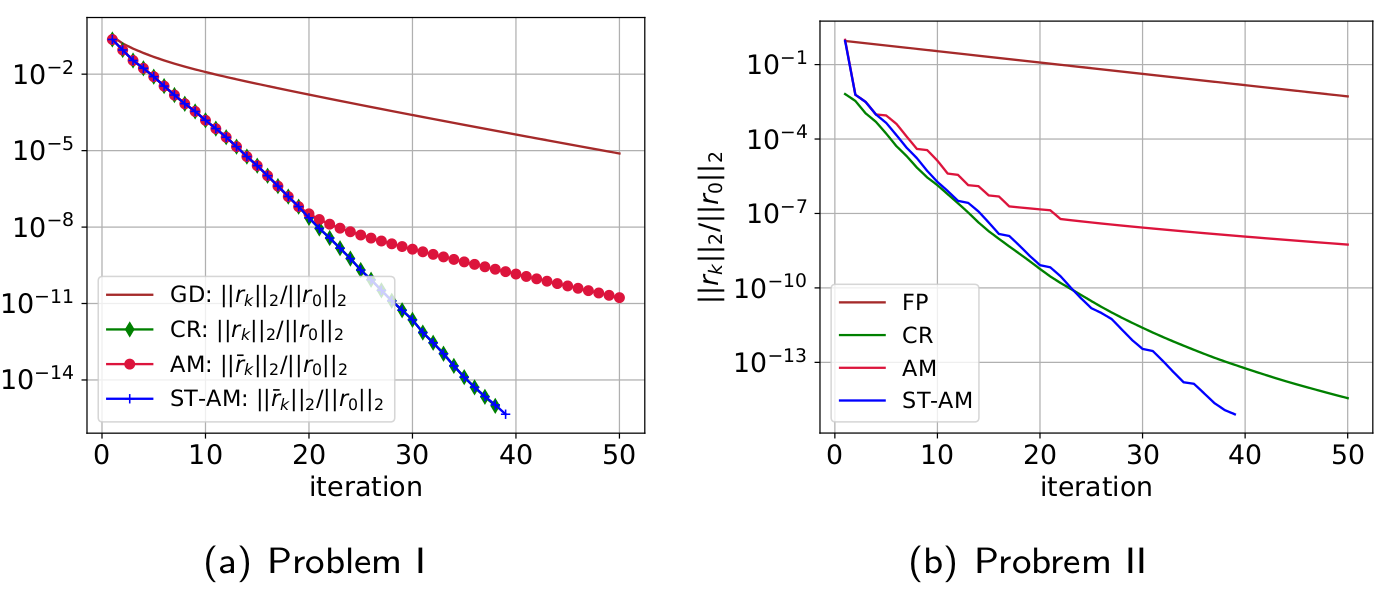The numerical results conform to our theorems (Problem I: a strongly convex quadratic optimization; Problem II: a nonsymmetric linear system). It also shows that ST-AM has better numerical stability than the full-memory AM.

### The modified ST-AM

For solving general nonlinear fixed-point problems, we introduce some minor changes to the basic ST-AM and obtain the modified version. The modified ST-AM (MST-AM) has improved local linear convergence.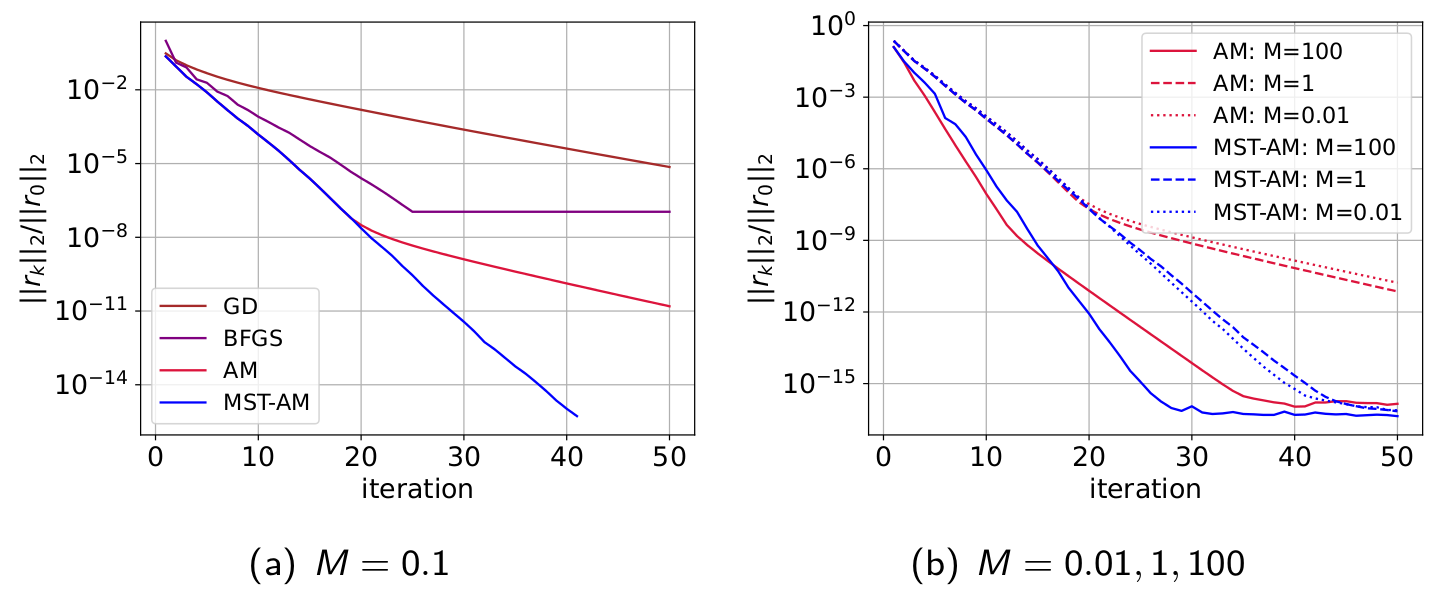The tests about the cubic-regularized quadratic optimization demonstrate the fast convergence of MST-AM.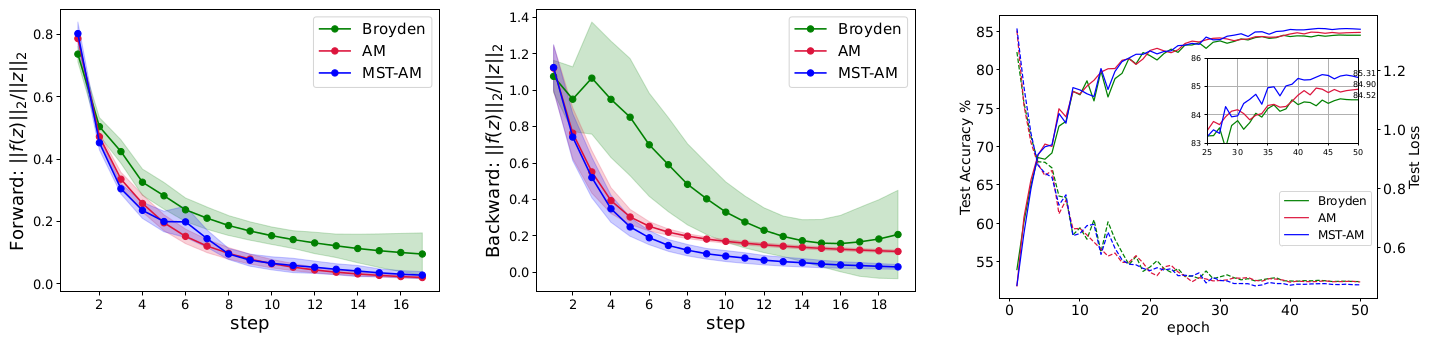The MST-AM is also comparable to AM and the Broyden’s method for solving the root-finding problems in the multiscale deep equilibrium model.

### The regularized ST-AM

To solve stochastic optimization, we introduce a regularized short-term recurrence form to the basic ST-AM. We also incorporate the damped projection and adaptive regularization techniques from stochastic Anderson mixing (SAM) (Wei et al., 2021) into ST-AM. The resulting method is the regularized ST-AM (RST-AM). RST-AM has global convergence for stochastic optimization, and the iteration complexity is $$O(1/\epsilon^2)$$.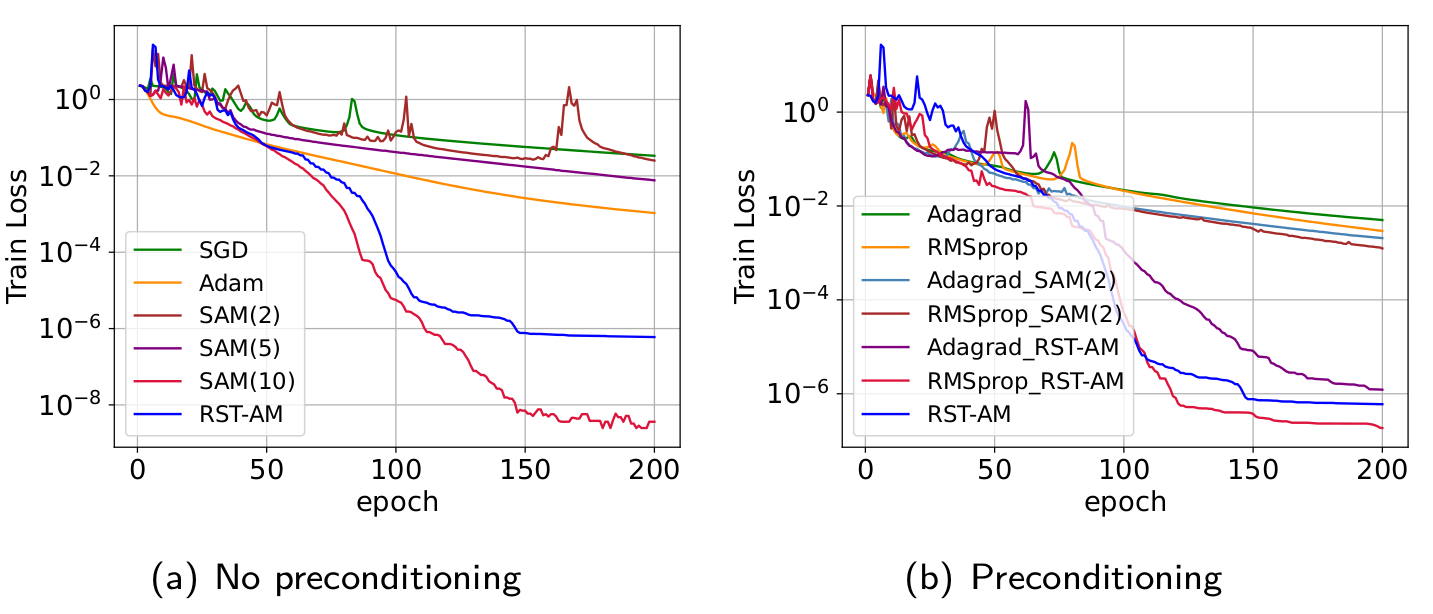For the full-batch training of a simple convolutional neural network (CNN) on MNIST dataset, the RST-AM can close the gap of the long-memory method (SAM(10)) and the short-memory methods (SAM(2), SAM(5)). The preconditioning is also helpful for the RST-AM.

The experiments of the mini-batch neural networks training include image classification on CIFAR, language model on Penn Treebank, adversarial training, and training a generative adversarial networks.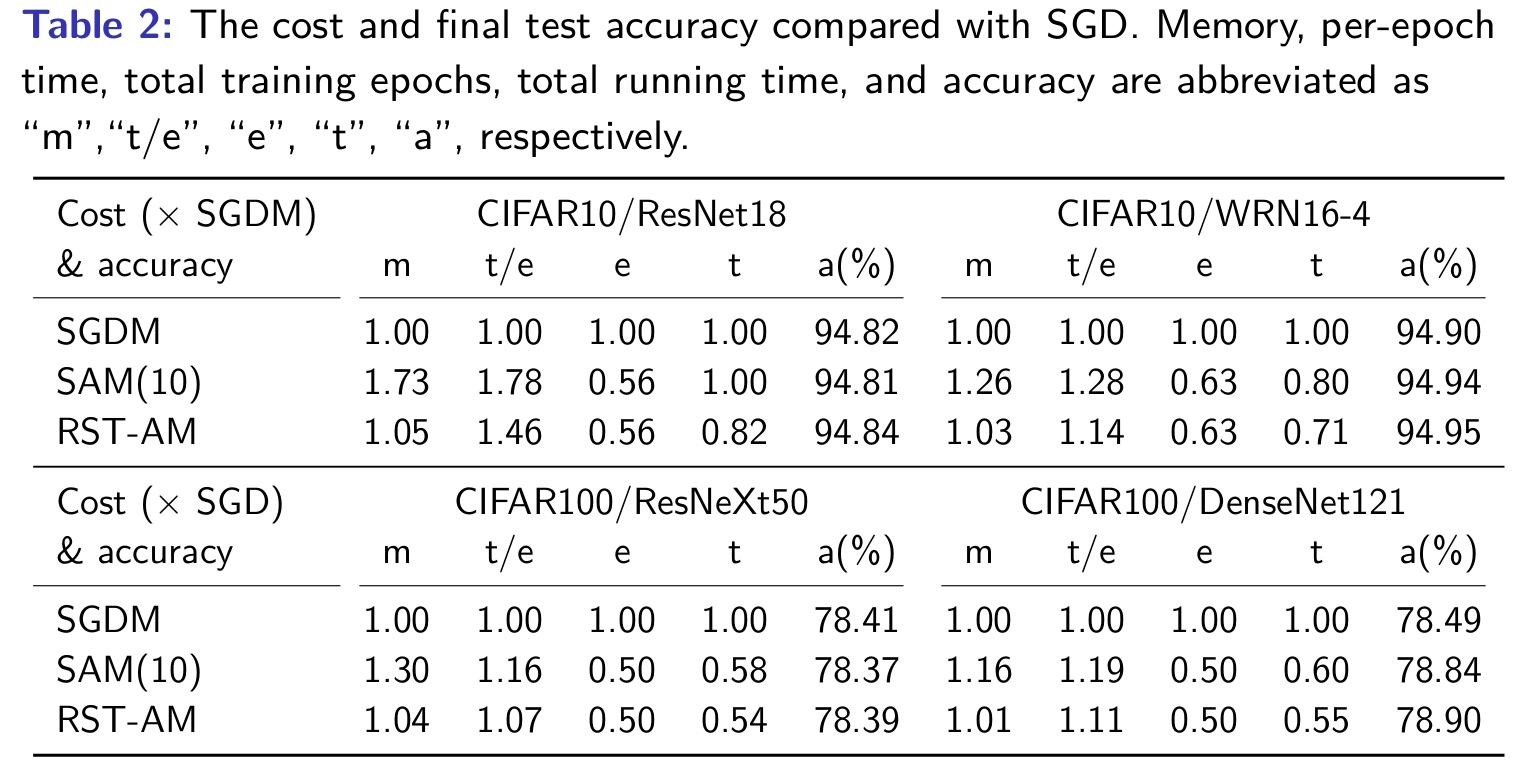We report the results of the tests on CIFAR here. The numerical results demonstrate that the regularized ST-AM outperforms many existing optimizers while being more memory-efficient than stochastic Anderson mixing. More experimental results can be found in the paper.

## Implementation

Here, we give a discussion about the implementation of our methods.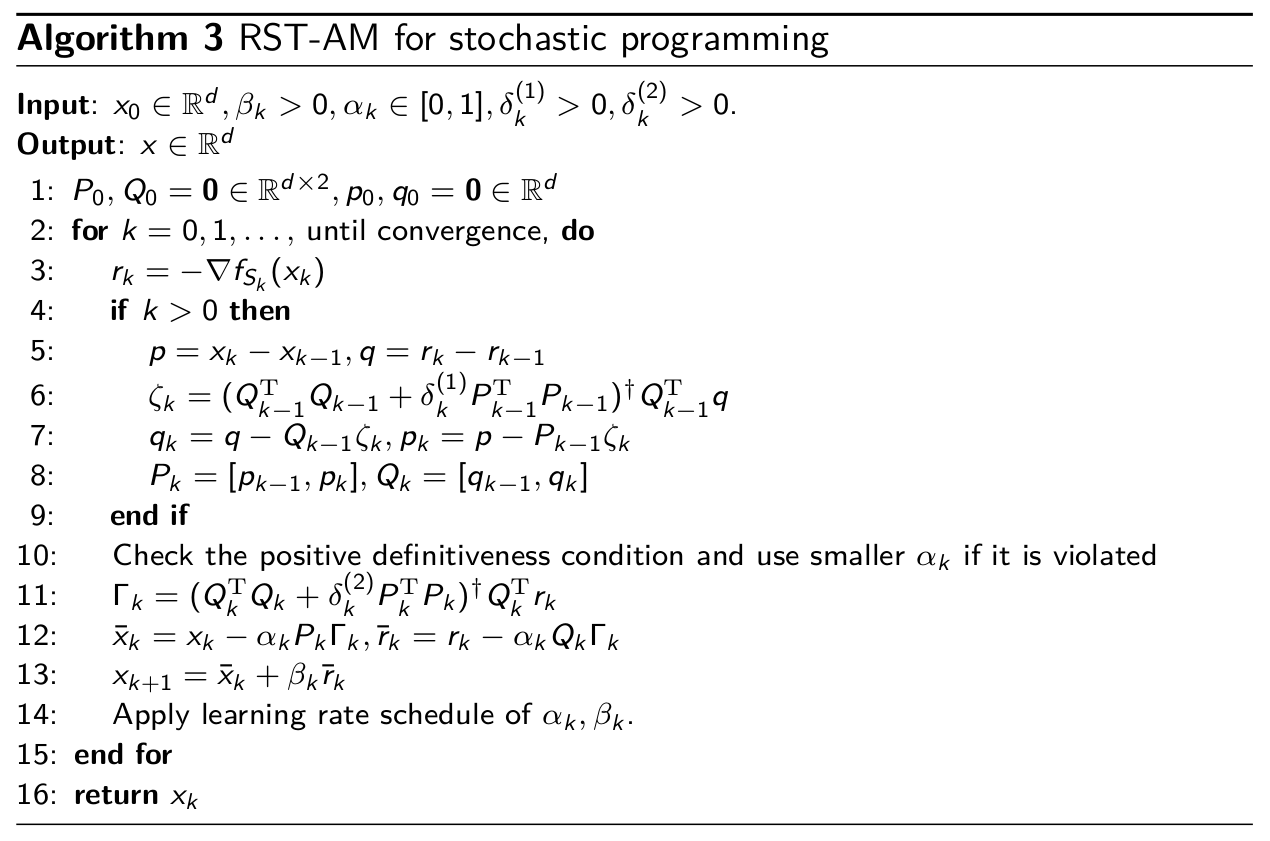Algorithm 3 describes the details of the RST-AM. When setting $$\delta_k^{(1)} = 0$$, $$\delta_k^{(2)} = 0$$ and $$\alpha_k = 1$$, it is reduced to the ST-AM/MST-AM method. Note that we need to use two matrices $$P, Q \in \mathbb{R}^{d\times 2}$$ to store $$P_k$$ and $$Q_k$$ during the iterations. So this additional memory cost of RST-AM compared with SGD is $$4d$$. For the stochastic Anderson mixing, this cost is $2md$, where $m=10$ in the experiments.

The choices of $$\delta_k^{(1)}$$ and $$\delta_k^{(2)}$$ are

$\delta_k^{(1)} = \frac{c_1\|r_k\|_2^2}{\|\delta x_{k-1}\|_2^2+\epsilon_0}, \delta_k^{(2)} = \max\{ \frac{c_2\|r_k\|_2^2}{\|p_k\|_2^2+\epsilon_0}, C\beta_k^{-2}\}$

where $$c_1 = 1$$, $$c_2 = 1\times 10^{-7}$$, $$\epsilon_0 = 1\times 10^{-8}$$, and $$C=0$$ in our implementation. We found that only $$c_1$$ and $$c_2$$ need to be carefully tuned in the experiments. Also, the setting that $$c_1 = 1$$, $$c_2 = 1\times 10^{-7}$$ is quite robust in all of our tests, so this default setting works well. The Line 10 is to guarantee the searching direction is a descent function. In practice, we check $$(x_{k+1}-x_k)^\mathrm{T}r_k$$ as a simplification. The $$\alpha_k$$ and $$\beta_k$$ are similar to the learning rate in SGD. Hence, the learning rate schedule directly acts on $$\alpha_k$$ and $$\beta_k$$.

## Conclusion

To address the memory issue of Anderson mixing, we develop a novel class of short-term recurrence Anderson mixing (ST-AM) methods and test it on various applications, including solving linear and nonlinear problems, deterministic and stochastic optimization. We give a complete theoretical analysis of the proposed methods. It is expected that the ST-AM methods can be applied to solve more challenging problems in scientific computing and machine learning, where the high dimensionality prohibits the applications of the classical second-order methods.